# 面向对象三大特征1148-张同学

,

## 方法没有重载

// An highlighted block
class Person:
def say1(self):
print("hello")

def say1(self):
print("hi")

p1=Person()
p1.say1()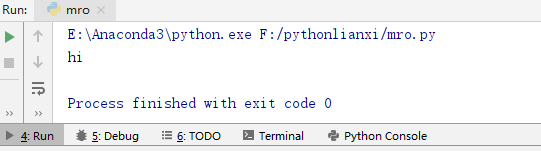## 方法的动态性

python是动态语音，可以动态的为类添加新方法，或者动态的修改类的已有的方法。

// An highlighted block
'''方法的动态性'''
class Person:

def work(self):
print("好好学习")

def play_game(s):
print("{0}在玩游戏".format(s))

Person.play=play_game
p=Person()           #Persom.play(p)
p.work()
p.play()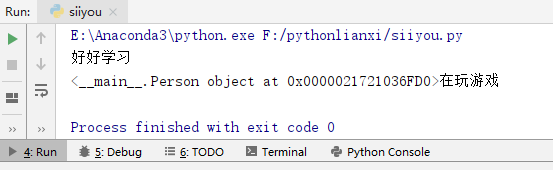## 私有属性和私有方法(实现封装)

python对于类的成员没有严格的访问控制限制，这与其他面向对象语言有区别。关于私有属性和私有方法，有如下要点：

1.通常约定，两个下划线开头的属性是私有的，其他的是公有的
2. 类内部可以访问私有属性(方法)
3.类外部不能通过访问私有属性(方法)
4.类外部可以通过“_类名__私有属性(方法)名”访问私有属性(方法)

// An highlighted block
'''测试私有属性，私有方法'''

class Employee:

def __init__(self,name,age):
self.name=name
self.__age=age              #设置私有属性

def __work(self):
print("好好学习")            #私有方法
print("年龄:{0}".format(self.__age))   #类内部调用

e = Employee("高琪",24)

print(e.name)
print(e._Employee__age)
print(dir(e))
e._Employee__work()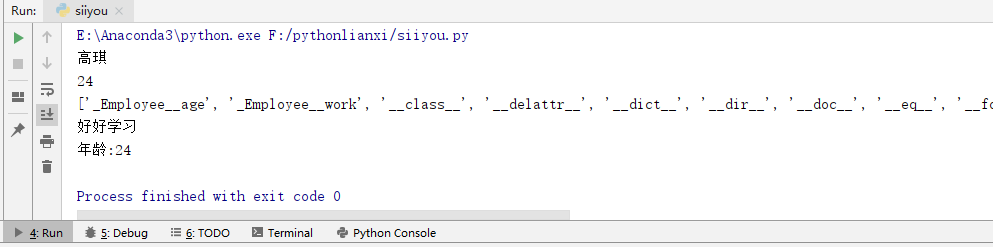## 面向对象三大特征介绍

1. 封装(隐藏)
隐藏对象的属性和实现细节，只对外提供必要的方法，相当于“细节封装起来”，只对外暴露“相关调用方法”
2. 继承
继承可以让子类具有父类的特性，提高了代码的重用性
从设计上是一种增量进化，原有父类设计不变的情况下，可以增加新的功能，或者改进已有的算法
3. 多态
多态指的是同一种方法调用由于对象不同会产生不同的行为。

#### 继承

class 子类类名(父类1[，父类2，…])
类体

// An highlighted block
'''继承'''
class Person:

def __init__(self,name,age):
self.name=name
self.__age=age

def say_age(self):
print(self.name,"年龄是：",self.__age)

class Student(Person):

def __init__(self,name,age,score): #必须显示的去调用父类初始化方法，不然解释器不会去调用
self.score=score
Person.__init__(self,name,age)

print(Student.mro())            #继承关系

s=Student("一个小白的日常",24,80)
s.say_age()
print(s.name)
print(dir(s))
print(s._Person__age)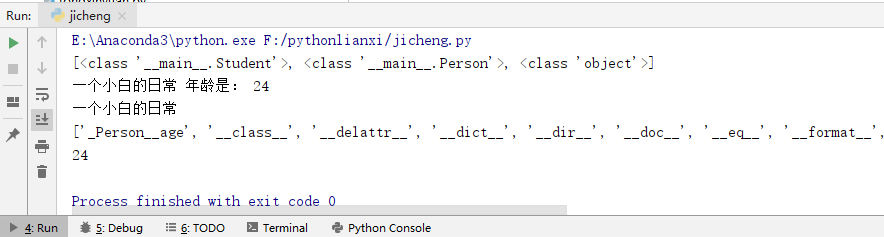1. 成员继承：子类继承了父类除构造方法之外的所有成员
2. 方法重写：子类可以重新定义父类中的方法，这样就会覆盖父类的方法。
// An highlighted block
'''类的继承和成员'''
class Person:

def __init__(self,name,age):
self.name=name
self.__age=age

def say_age(self):
print("年龄是：",self.__age)

def say_introduce(self):
print("名字是{0}：".format(self.name))

class Student(Person):

def __init__(self,name,age,score):
Person.__init__(self,name,age)  #必须显示的去调用父类初始化方法，不然解释器不会去调用
self.score = score

def say_introduce(self):
'''重写的父类的方法'''
print("我的名字是: {0}".format(self.name))

s=Student("一个小白的日常",24,80)
s.say_age()
s.say_introduce()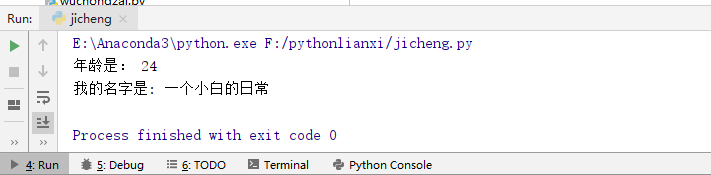super()获得父类定义
super()获得父类定义，不是父类对象

// An highlighted block
'''测试super()，代表父类的定义，而不是父类的对象'''
class A:

def say(self):
print("A:",self)

class B(A):

def say(self):
super().say()
print("B",self)

B().say()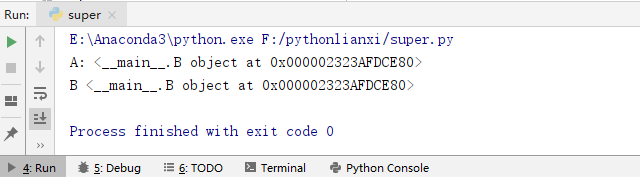#### 多重继承

// An highlighted block
'''测试多重继承的方法解析顺序'''
class A:
def aa(self):
print("aa")
def  say(self):
print("say AAA")

class B:
def bb(self):
print("bb")
def say(self):
print("say BBB")

class C(B,A):
def cc(self):
print("cc")

c=C()
c.cc()
c.bb()
c.aa()
c.say()         #解析器寻找方法是“从左到右”的方式寻找，此时会执行B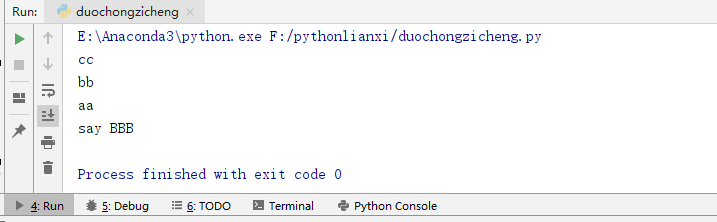#### 多态

1. 多态是方法的多态，属性没有多态
2. 多态的存在有两个必要条件：继承、方法重写
// An highlighted block
'''多态'''
class Man:
def eat(self):
print("吃饭")

class Chinese(Man):
def eat(self):
print("中国人用筷子吃饭")
class English(Man):
def eat(self):
print("英国人用叉子吃饭")
class Indian(Man):
def eat(self):
print("印度人用右手吃饭")

def manEat(n):
if isinstance(n,Man):
n.eat()               #多态，一个方法调用，根据对象的不同调用不同的方法
else:
print("不能吃饭")

manEat(Chinese())
manEat(English())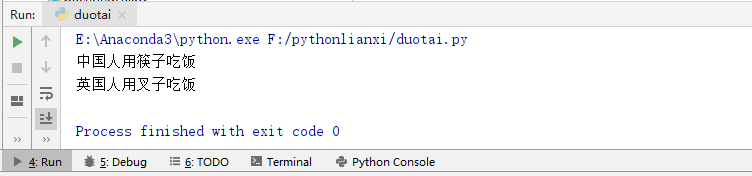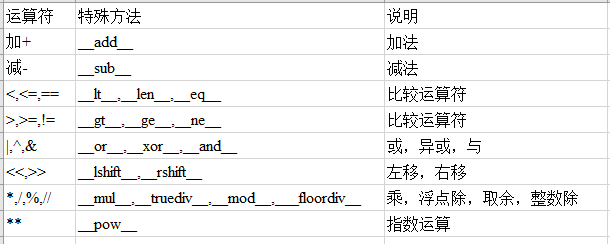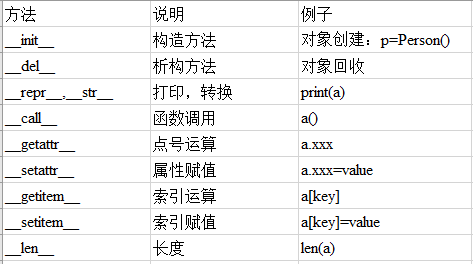// An highlighted block
'''特殊方法和运算符重载'''
class Person:
def __init__(self,name):
self.name=name

if isinstance(other,Person):
return "{0}--{1}".format(self.name,other.name)
else:
return "不是同类对象，不能相加"

p1=Person("高一")
p2=Person("高二")
x=p1+p2
print(x)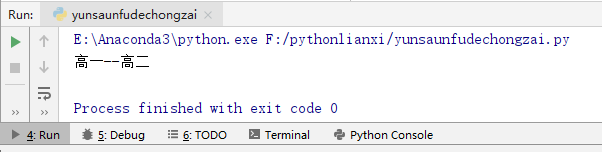python对象中包含了很多下划线开始和结束的属性，这些是特殊属性，有特殊用法。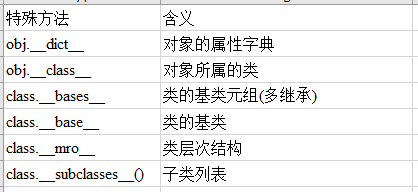// An highlighted block
'''特殊属性'''
class A:
def aa(self):
print("aa")
def  say(self):
print("say AAA")

class B:
def bb(self):
print("bb")
def say(self):
print("say BBB")

class C(B,A):

def __init__(self,nn):
self.nn=nn

def cc(self):
print("cc")

c=C(3)
c.say()         #解析器寻找方法是“从左到右”的方式寻找，此时会执行B
print(dir(c))
print(c.__dict__)
print(c.__class__)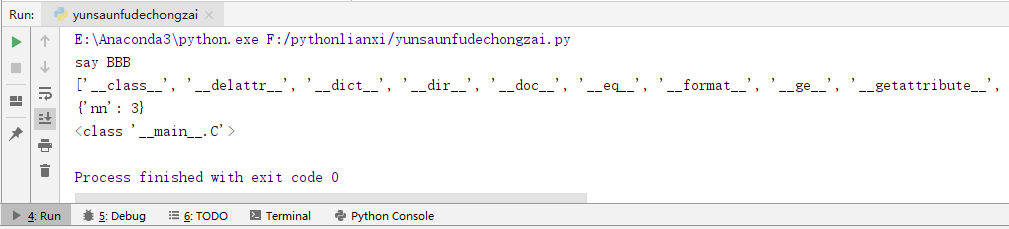## 对象的浅拷贝和深拷贝

python拷贝一般都是浅拷贝，拷贝时，对象包含的子对象内容不拷贝。因此，源对象和拷贝对象会引用同一个对象。

// An highlighted block
import copy
class MobilePhone:
def __init__(self,cpu,screen):
self.cpu=cpu
self.screen=screen

class CPU:
def calculate(self):
print("cpu对象：",self)

class Screen:
def show(self):
print("screen对象: ",self)

#"测试变量赋值"
c1=CPU()
c2=c1
print(c1)
print(c2)
print("**********")

#"测试浅赋值"
s1=Screen()
m1=MobilePhone(c1,s1)
m2 = copy.copy(m1)
print("测试浅拷贝")
print(m1,m1.cpu,m1.screen)
print(m2,m2.cpu,m2.screen)
print("**********")

#测试深赋值
m3=copy.deepcopy(m1)
print("测试深拷贝")
print(m1,m1.cpu,m1.screen)
print(m3,m3.cpu,m3.screen)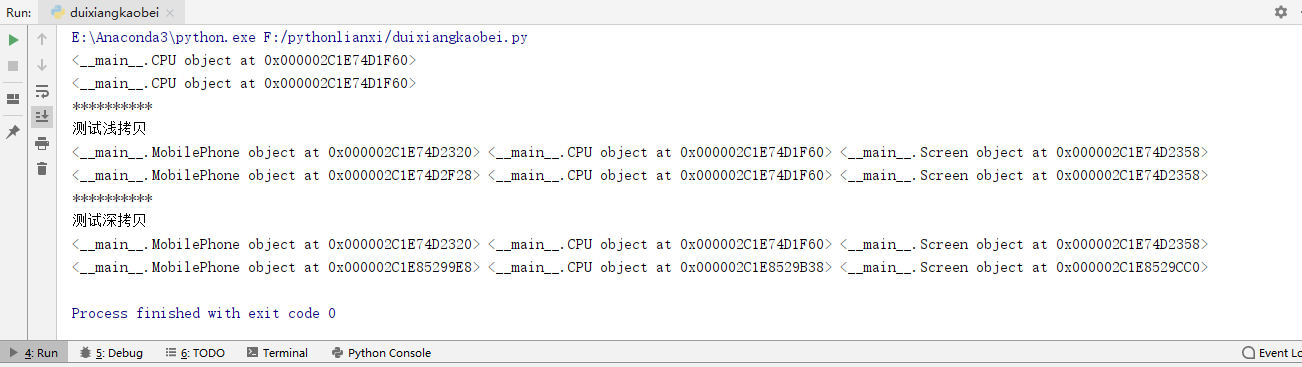## 组合

// An highlighted block
'''组合'''
class MobilePhone:
def __init__(self,cpu,screen):
self.cpu=cpu
self.screen=screen

class CPU:
def calculate(self):
print("cpu对象：",self)

class Screen:
def show(self):
print("screen对象: ",self)

m=MobilePhone(CPU(),Screen())
m.cpu.calculate()
m.screen.show()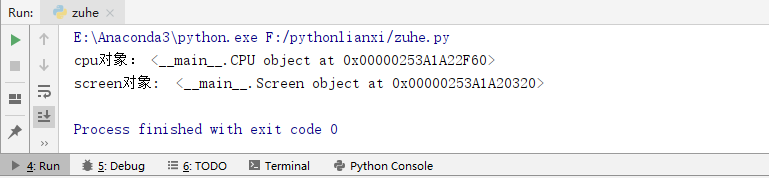### 评论 抢沙发### 觉得文章有用就打赏一下文章作者

#### 支付宝扫一扫打赏#### 微信扫一扫打赏Vieu3.3主题Q Q 登 录